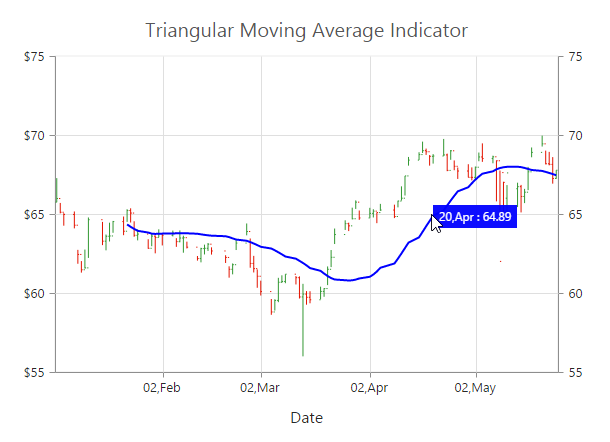# Technical Indicators

13 Jun 202312 minutes to read

EjChart control supports 10 types of technical indicators.

## Bind data to render the indicator

You can bind the series DataSource to the indicator by setting the specific series name to the indicator by using the Indicators.SeriesName property.

• CSHTML
• ``````@(Html.EJ().Chart("chartContainer")

// ...
//Initializing Series
.Series(sr =>
{
sr
.XName("XDate").High("High").Low("Low").Open("Open").Close("Close")
//Set name to series
.Name("Hilo")
})
//Initializing Indicators
.Indicators(ind => { ind
//Set HiLo series dataSource to indicator using seriesName
})
//...
)``````
• CSHARP
• ``````//// Create dataSource to chart
List<ChartData> data = new List<ChartData>();
data.Add(new ChartData("2010/7/1", 38, 10, 58, 27));
data.Add(new ChartData("2010/7/8", 28, 15, 48, 58));
data.Add(new ChartData("2010/7/9", 54, 35, 85, 98));
data.Add(new ChartData("2010/7/10", 52, 21, 38, 85));
///...
ViewBag.ChartData = data;``````

Also, you can add data to the indicator directly by using the DataSource option of the indicator.

• CSHTML
• ``````@(Html.EJ().Chart("chartContainer")

// ...

//Initializing Indicators
.Indicators(ind => { ind
.XName("XDate").High("High").Low("Low").Open("Open").Close("Close")
})
//...
)``````

## Indicator Types

### Accumulation Distribution

To create an Accumulation Distribution indicator, set the Indicators.Type as AccumulationDistribution. Accumulation Distribution require Volume field additionally with the DataSource to calculate the signal line.

• CSHTML
• ``````@(Html.EJ().Chart("chartContainer")

// ...
//Initializing Series
.Series(sr =>
{
sr
.XName("XDate").High("High").Low("Low").Open("Open").Close("Close").Volume("Volume")
//Set name to series
.Name("Hilo")
})
//Initializing Indicators
.Indicators(ind => { ind
//Set indicator type
.Type(ChartIndicatorType.AccumulationDistribution)
})
//...
)``````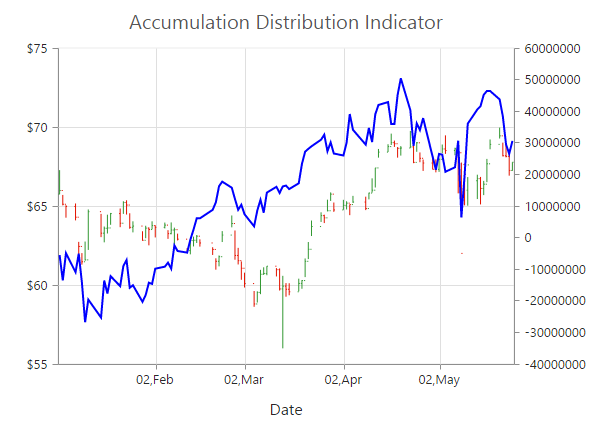### Average True Range (ATR)

You can create an ATR indicator by setting the Indicators.Type as ATR in the Indicators.

• CSHTML
• ``````@(Html.EJ().Chart("chartContainer")

// ...
//Initializing Series
.Series(sr =>
{
//...
})
//Initializing Indicators
.Indicators(ind => { ind
//Set indicator type
})
//...
)``````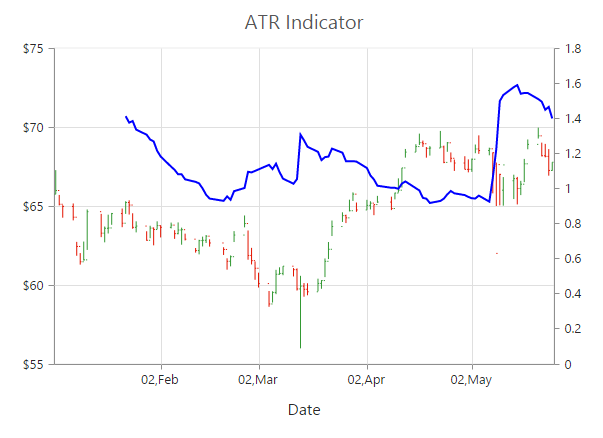### Bollinger Band

Bollinger Band indicator is created by setting the Indicators.Type as BollingerBand. It contains three lines, namely upper band, lower band and signal line. Bollinger Band default value of the Period is 14 and StandardDeviations is 2.

• CSHTML
• ``````@(Html.EJ().Chart("chartContainer")

// ...
//Initializing Series
.Series(sr =>
{
//...
})
//Initializing Indicators
.Indicators(ind => { ind
//Set indicator type
})
//...
)``````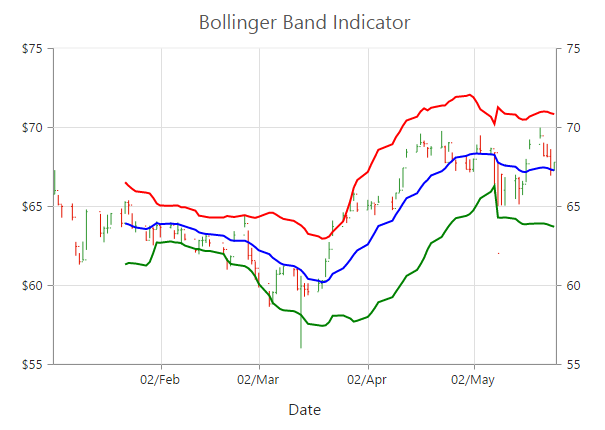### Exponential Moving Average (EMA)

To render an EMA indicator, you have to set the Indicators.Type as EMA.

• CSHTML
• ``````@(Html.EJ().Chart("chartContainer")

// ...
//Initializing Series
.Series(sr =>
{
//...
})
//Initializing Indicators
.Indicators(ind => { ind
//Set indicator type
})
//...
)``````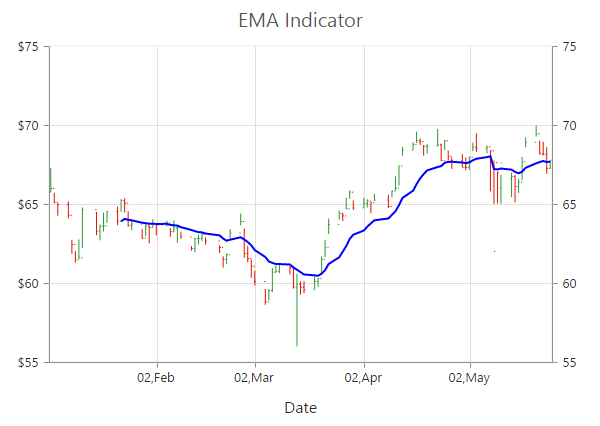### Momentum

Momentum Technical indicator is created by setting the Indicators.Type as Momentum. The momentum indicator renders two lines, namely upper band and signal line. Upper band always rendered at the value 100 and the signal line is calculated based on the momentum of the data.

• CSHTML
• ``````@(Html.EJ().Chart("chartContainer")

// ...
//Initializing Series
.Series(sr =>
{
//...
})
//Initializing Indicators
.Indicators(ind => { ind
//Set indicator type
})
//...
)``````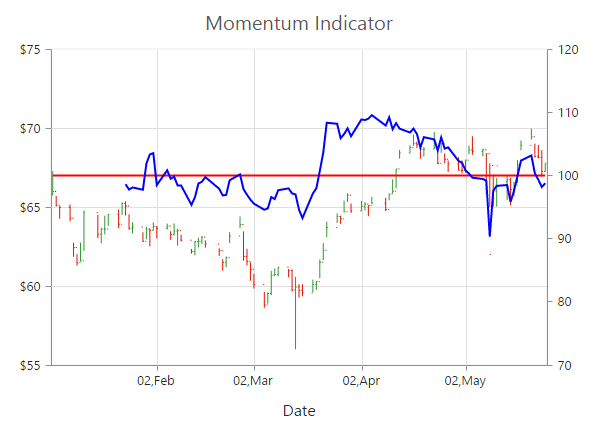### Moving Average Convergence Divergence (MACD)

To render an MACD indicator, you have to set the Indicators.Type as MACD. MACD indicator contains MACD line, Signal line and Histogram column. Histogram is used to differentiate MACD and signal line.

• CSHTML
• ``````@(Html.EJ().Chart("chartContainer")

// ...
//Initializing Series
.Series(sr =>
{
//...
})
//Initializing Indicators
.Indicators(ind => { ind
//Set indicator type
})
//...
)``````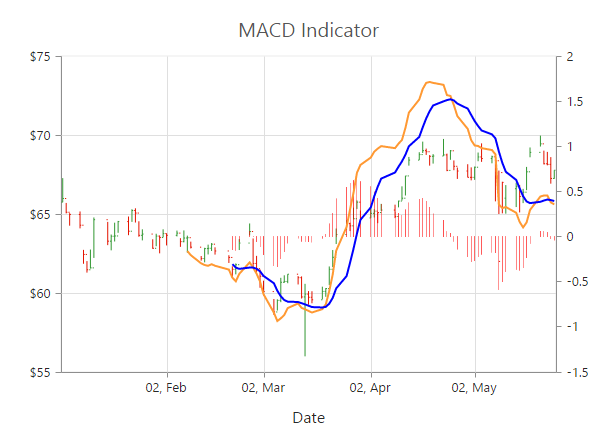#### MacdType

By using the MacdType enumeration property, you can change the MACD rendering as Line, Histogram or Both.

• CSHTML
• ``````@(Html.EJ().Chart("chartContainer")

// ...
//Initializing Series
.Series(sr =>
{
//...
})
//Initializing Indicators
.Indicators(ind => { ind
.Type(ChartIndicatorType.MACD)
//Set MACD draw type
.MacdType(MacdType.Histogram)
})
//...
)``````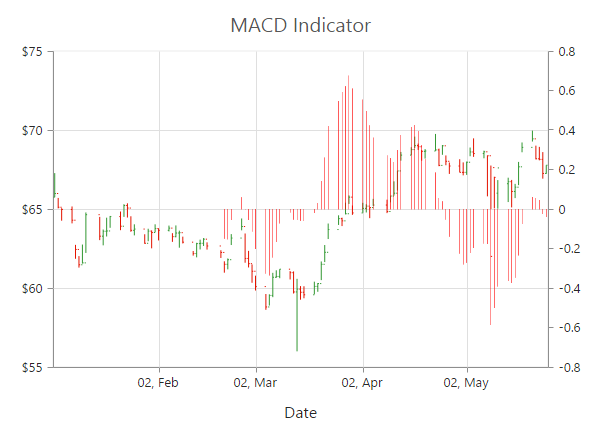### Relative Strength Index (RSI)

To render the RSI indicator, set the Indicators.Type as RSI. It contains three lines, namely upper band, lower band and signal line. Upper and lower band always render at value 70 and 30 respectively and signal line is calculated based on the RSI formula.

• CSHTML
• ``````@(Html.EJ().Chart("chartContainer")

// ...
//Initializing Series
.Series(sr =>
{
//...
})
//Initializing Indicators
.Indicators(ind => { ind
//Set indicator type
.Type(ChartIndicatorType.RSI)
})
//...
)``````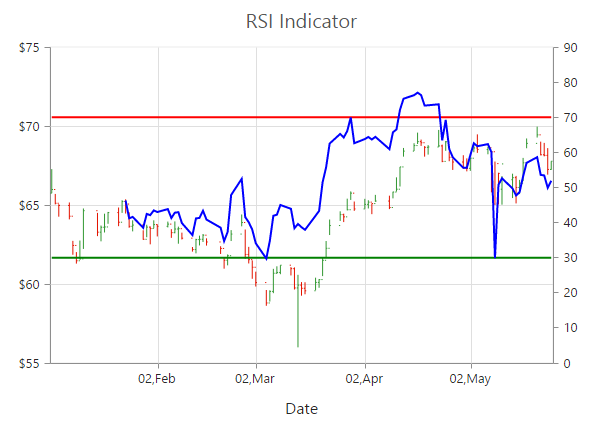### Simple Moving Average (SMA)

To render the SMA indicator, you should specify the Indicators.Type as SMA.

• CSHTML
• ``````@(Html.EJ().Chart("chartContainer")

// ...
//Initializing Series
.Series(sr =>
{
//...
})
//Initializing Indicators
.Indicators(ind => { ind
//Set indicator type
.Type(ChartIndicatorType.SMA)
})
//...
)``````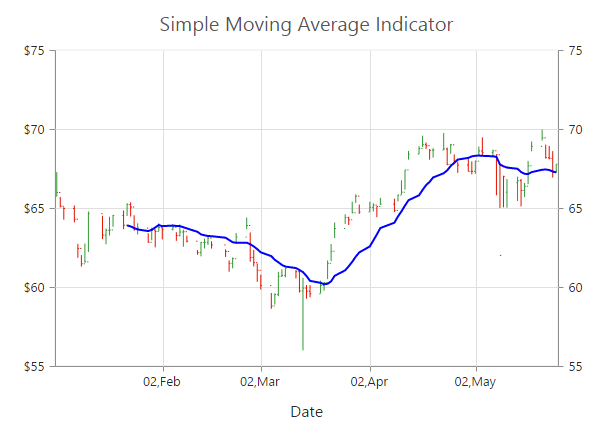### Stochastic

For the Stochastic indicator, you need to set the Indicators.Type as Stochastic. The Stochastic indicator renders four lines namely, upper line, lower line, stochastic line and the signal line. Upper line always rendered at value 80 and the lower line is rendered at value 20. Stochastic and Signal Lines are calculated based on the stochastic formula.

• CSHTML
• ``````@(Html.EJ().Chart("chartContainer")

// ...
//Initializing Series
.Series(sr =>
{
//...
})
//Initializing Indicators
.Indicators(ind => { ind
//Set indicator type
.Type(ChartIndicatorType.Stochastic)
})
//...
)``````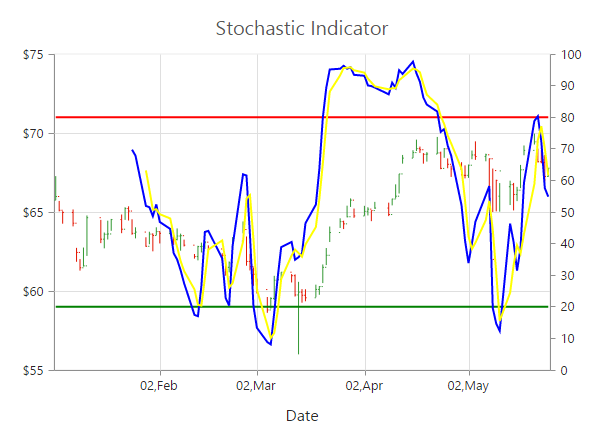### Triangular Moving Average (TMA)

To render the TMA indicator, you should specify the Indicators.Type as TMA.

• CSHTML
• ``````@(Html.EJ().Chart("chartContainer")

// ...
//Initializing Series
.Series(sr =>
{
//...
})
//Initializing Indicators
.Indicators(ind => { ind
//Set indicator type
.Type(ChartIndicatorType.TMA)
})
//...
)``````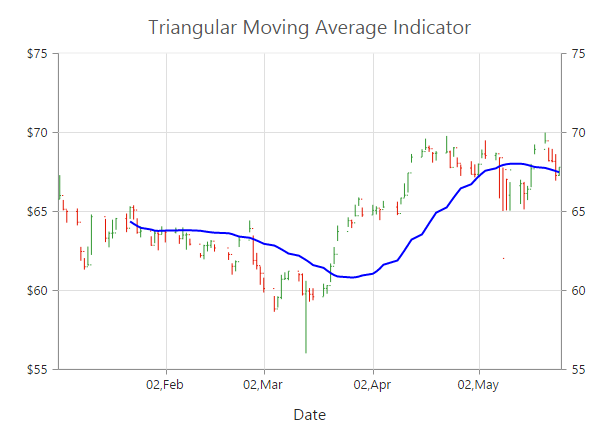## Enable Tooltip

To display the indicator tooltip, use Visible option of the Indicators.Tooltip. Also, you can change and customize the tooltip color, border, format and font properties similar to the series tooltip.

• CSHTML
• ``````@(Html.EJ().Chart("chartContainer")

// ...
//Initializing Series
.Series(sr =>
{
//...
})
//Initializing Indicators
.Indicators(ind => { ind
//...
.Tooltip(tooltip=>tooltip.
//Enable tooltip for indicator
Visible(true))